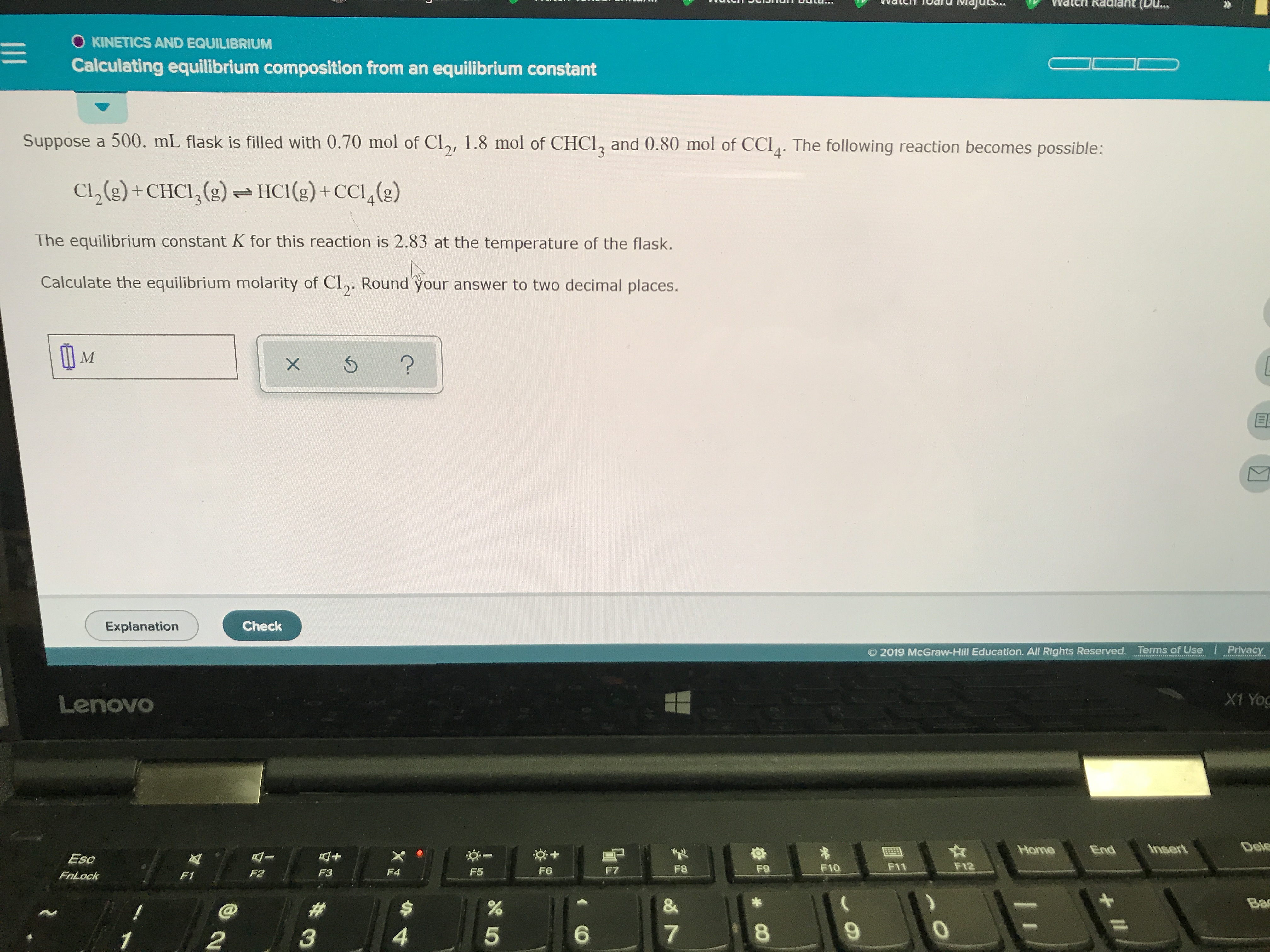# Suppose a 500.0 mL flask is filled with 0.70 mol of Cl2, 1.8 mol of CH3Cl and 0.80 mol of CCl4. The following reaction becomes possible Cl2(g) + CJHCl3(g) <-> HCl(g) + CCl4(g). The equilibrium constant K for this reaction is 2.83 at the temperature of the flask. Calculate the equilibrium molarity of Cl2. Round your answer to 2 decimal places.